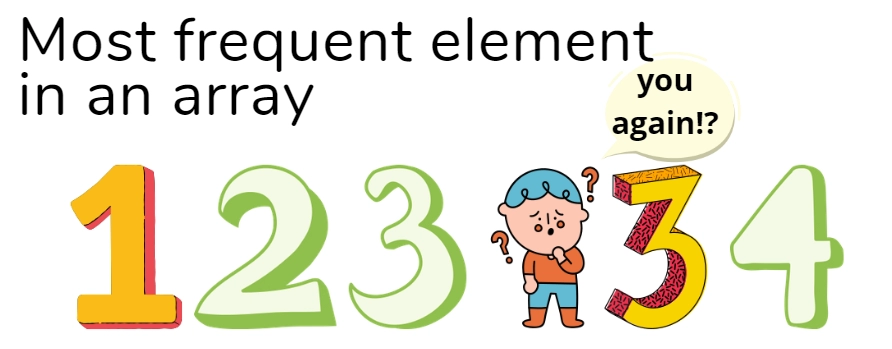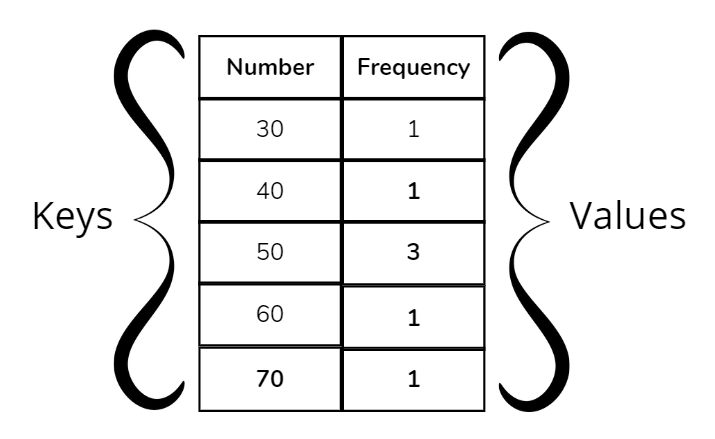New update is available. Click here to update.
Last Updated: Jun 30, 2023
Easy

# Most frequent element in an array

## Introduction

In this article, we will discuss the problem named to find the most frequent element in an array. Before jumping on to the approach to the problem, let us first understand the problem.### Problem Statement

Here we need to find the element that occurs the most frequently in the n-element size array Arry[], that is, the element that occurs the most frequently overall. There will always be at least one repeating element.

Example :

The maximum frequency in the Arry1 is 2, which appears two times.

Here as you can see the maximum frequency in the Arry2 is 3, which appears three times in the given array.

### Efficient solutions and brute force🤔

In the below section, these possible solutions for resolving this problem will be discussed:

1. Using nested loops to calculate frequency using brute force.

2. Hash table usage to find the frequency of elements using a hash table.## Approach-1: Brute Force Approach

In this approach we will find the repeated element by running two loops.

•  First all elements are chosen one by one by the outer loop.
• The inner loop calculates the frequency of the chosen element.
• And then it compares it with the maximum value obtained so far.

## Implementation

``````#include <bits/stdc++.h>
using namespace std;
int mostFreqnt(int * arry, int n) {
int max_count = 0;
int maxfreqelement;
for (int i = 0; i < n; i++) {
int count = 0;
for (int j = 0; j < n; j++) {
if (arry[i] == arry[j])
count++;
}
if (count > max_count) {
max_count = count;
maxfreqelement = arry[i];
}
}
return maxfreqelement;
}
int main() {
int arry[] = {4,5,3,4,5, 3,3 };
int n = sizeof(arry) / sizeof(arry);
cout << mostFreqnt(arry, n);
return 0;
}``````

Output :

This solution has a time complexity of  O(n2) Since there are two loops that run from i=0 to i=n, omitting the numbers in a visited array for which we already know the frequency will decrease the time complexity.

## Approach-2: Hashing

Use of hashing is an effective solution. As key-value pairs, we store elements and their frequency counts in a hash table.The hash table is then traversed, and the key with the maximum value is printed.

Now, let us quickly look at its Implementation:

## Implementation in C++

``````#include <bits/stdc++.h>
using namespace std;

int mostFrequentelement(int arry[], int n) {
// Inserting all the elements.
unordered_map < int, int > hash;
for (int i = 0; i < n; i++)
hash[arry[i]]++;

// finding max frequency
int maxcount = 0, res = -1;
for (auto i: hash) {
if (maxcount < i.second) {
res = i.first;
maxcount = i.second;
}
}

return res;
}

// driver program
int main() {
int arry[] = {50,60,30,50,50,70,40};
int n = sizeof(arry) / sizeof(arry);
cout << mostFrequentelement(arry, n);
return 0;
}``````

Output :

This solution has a time complexity of O(n)) as the for loop will run n times and auxiliary space of O(n).

You can also read about the Longest Consecutive Sequence, Fibonacci Series in Python

## Implementation in Java

Here is the  java program to find the most frequent element in an array using a hash function.

``````import java.util.HashMap;
import java.util.Map;
import java.util.Map.Entry;
class Main {

static int mostFrequent(int array[], int n) {

// Insert all elements in hash
Map < Integer, Integer > hm =
new HashMap < Integer, Integer > ();

for (int i = 0; i < n; i++) {
int key = array[i];
if (hm.containsKey(key)) {
int freq = hm.get(key);
freq++;
hm.put(key, freq);
} else {
hm.put(key, 1);
}
}

// finding max frequency.
int max_count = 0, res = -1;

for (Entry < Integer, Integer > val: hm.entrySet()) {
if (max_count < val.getValue()) {
res = val.getKey();
max_count = val.getValue();
}
}
System.out.println("The most frequent element in the array is");
return res;
}
// Driver code
public static void main(String[] args) {

int array[] = {40,40,30,40,50,3,30};
int n = array.length;
System.out.println(mostFrequent(array, n));
}
}``````

Output :

This solution also has a time complexity of O(n)) as the for loop here will run n times and auxiliary space of O(n).

## Implementation in Python3

Now let’s discuss the Python3 program to find the most  frequent element in an array.

``````import math as mt
def mostFrequent(array, n):
# Insert all elements in Hash.
Hash = dict()
for i in range(n):
if array[i] in Hash.keys():
Hash[array[i]] = 1
else:
Hash[array[i]] = 1
# find the max frequency
max_count = 0
res = -1
for i in Hash:
if max_count < Hash[i]:
res = i
max_count = Hash[i]
return res
# Driver Code
array = [20, 10, 40, 20, 80, 10, 20]
n = len(array)
print(mostFrequent(array, n))``````

Output :

This solution has a time complexity of O(n) as the for loop will run n times and auxiliary space of O(n).

### What is the number that occurs the most frequently?

The mode of a data set is the number that appears the most frequently. For instance, determine the mode of the numbers 2, 7, 13, and 2. While all the other numbers only appear once, the number 2 appears twice. Consequently, the answer is 2.

### In Python, how can I determine which elements are most frequent?

Use Python Counter, which provides a count for each element in a list. So, using the most common() method, we can easily identify the element that is used the most.

### How can you use C++ to identify a number's occurrences in an array?

std:: count() returns the number of times an element appears in a defined range. The number of elements in the range [first,last] that compare equal to val is returned.

### What is the built-in sort function's time complexity?

As most languages implement timsort, which is a hybrid of merge sort and insertion sort, the built-in sort function has a nlogn time complexity.

### Describe the frequency array.

A frequency array, also known as a frequency distribution, is an array of frequencies organised according to variable values. The individual frequency distributions that form the separate rows and columns of a bivariate frequency table are frequently referred to as an "array".

## Conclusion

In this article, we have discussed a simple and hashmap approach to find the most frequent element in an array. We hope that this article has helped you enhance your knowledge on finding the frequent elements. If you would like to learn more, check out our articles on arraysintroduction-to-hashingsort-the-given-array-after-sorting-each-number-individually and many more.

Check out the following problems -Refer to our guided paths on Coding Ninjas Studio to learn more about DSA, Competitive Programming, JavaScript, System Design, etc. Enrol in our courses and refer to the mock test and problems available, Take a look at the interview experiences and interview bundle for placement preparations.

Do upvote our blog to help other ninjas grow.

Happy Learning!